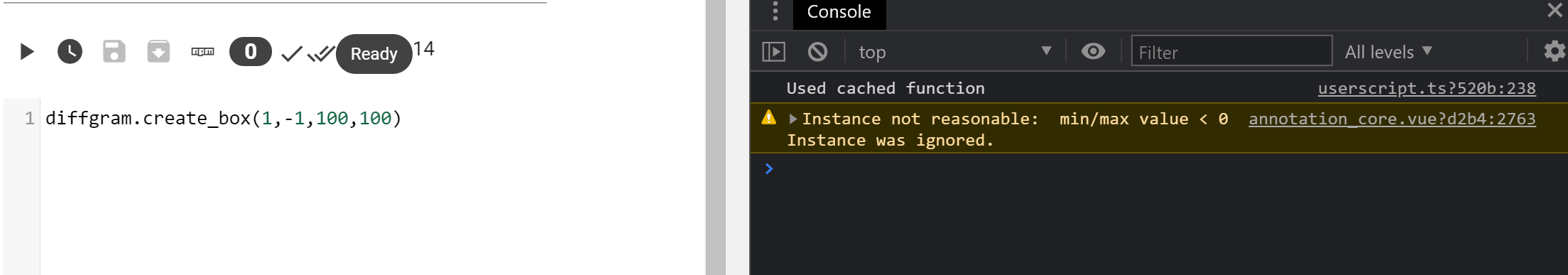# create_box

`create_box: function(x_min, y_min, x_max, y_max)`

# Description

Creates an instance of type `box` at the desired spatial coordinates.
The instance defaults to the current `label` the user has selected.

The function uses `check_reasonableness` and will warn if the instance is not reasonable (eg has negative values).

# Example

``````diffgram.create_box(0,0,100,100)
``````

# Example - Conversion

For example from using coco-ssd
They state that they have `bbox: [x, y, width, height]`. Here we convert `width` to x_max by doing `width - x`

``````diffgram.create_box(
prediction.bbox,
prediction.bbox,
prediction.bbox + prediction.bbox,
prediction.bbox + prediction.bbox)
}
``````

# Warnings

`Instance not reasonable`# Errors

Must have x_min, y_min, x_max, y_max